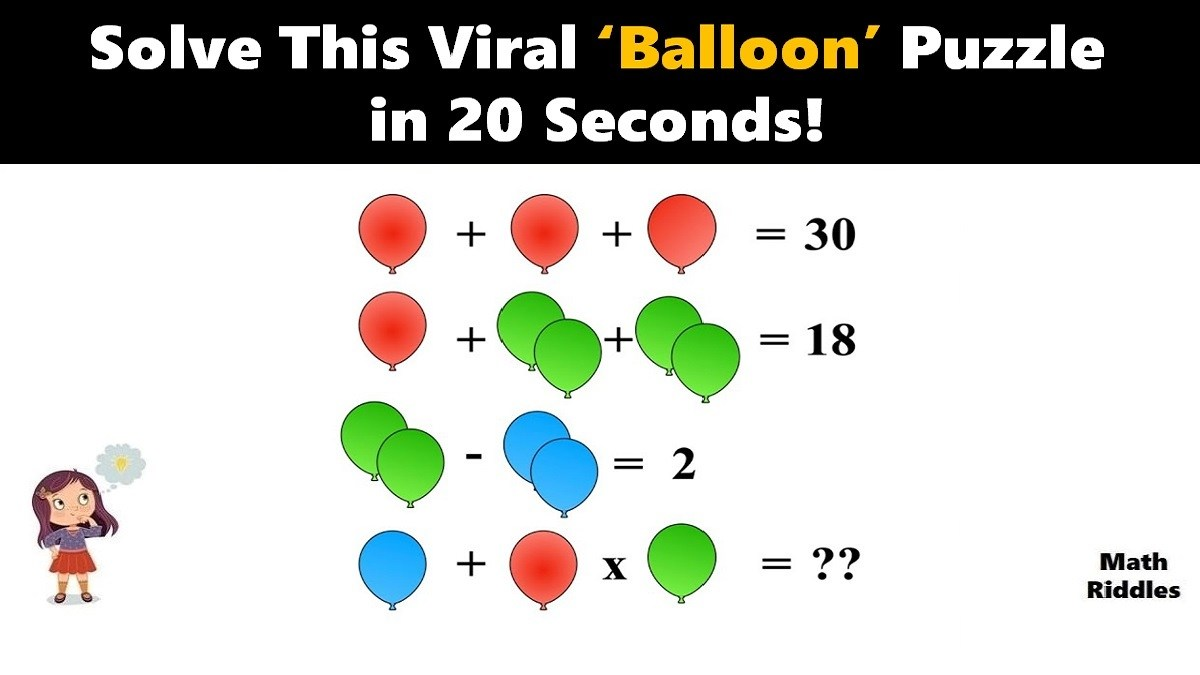# Math Riddles: Viral ‘Balloon’ Picture Puzzle, Only Genius Can Solve

Math Picture Puzzles: Find out how brilliant you are. Only 1% of people with a high IQ can solve this tricky ‘Balloon’ math picture puzzle in 20 seconds.Math Riddles: Viral ‘Balloon’ Picture Puzzle, Only Genius Can Solve

Math Riddles are so challenging, but that makes them worthwhile to solve. Math riddles are logical problems that require strong analytical abilities, high IQ, knowledge of math concepts, and good calculation skills. Math riddles have also been known to make Mathematics enjoyable for kids, students, and even adults to solve. Riddles, puzzles, and brain teasers can develop strategic thinking, logical reasoning, and problem-solving skills.

Today, we bring you another math picture puzzle that is all over social media. This only for genius ‘Balloon’ math picture puzzle has attracted millions of answers but very few got it right.

Can you solve this ‘Heart’ math picture puzzle? You have 20 seconds!

## Math Riddles: Balloon Puzzle with Answer

Math Picture Puzzle #1

Row 1: There are three red balloons which equals to 30.

So, we get 3 red balloons= 30, if we divide both sides by 3 then we get the result that 1 red balloon is equal to 10. So, we have 10 + 10 + 10 = 30. Now, we will use this value of 1 red balloon to solve next row of problem.

Row 2: There are 1 red balloon + 2 green balloons + 2 green balloons = 18

We will apply the value of 1 red balloon that we found in the row 1 equation. So, we get the following:

10 + 2 green balloons + 2 green balloons= 18

=> 4 green balloons = 8

=> 1 green balloon = 2

Row 3: There are 2 green balloons – 2 blue balloons = 2

So far, we have found that the value of 1 green balloon is 2. Hence, the value of 2 green balloons will be 4. Accordingly, we apply the value in the row 3 equation.

=> 4 – 2 blue balloons = 2

=> 2 blue balloons = 2

=> 1 blue balloon = 1

Row 4: There 1 blue balloon + 1 red balloon x 1 green balloon = ?

So far, we have ascertained that the value of 1 red balloon is 10, the value of 1 green balloon is 2, and the value of 1 blue balloon is 1. We will apply thee values accordingly to solve the final equation.

1 blue balloon + 1 red balloon x 1 green balloon = ?

=> 1 + 10 x 2 = ?

Apply BODMAS.

=> 1 + 20 = ?

=> 21

## Tell us in comments: Did you solve this math picture puzzle correctly in 20 seconds?

Check out more amazing tricky math puzzles!

Also Read: Math Riddles: Viral ‘Heart’ Picture Puzzle, Only Genius Can Solve